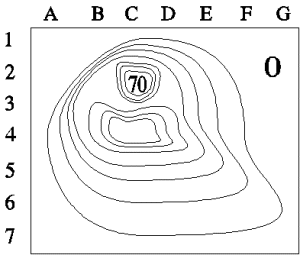• If you are citizen of an European Union member nation, you may not use this service unless you are at least 16 years old.

• Get control of your email attachments. Connect all your Gmail accounts and in less than 2 minutes, Dokkio will automatically organize your file attachments. You can also connect Dokkio to Drive, Dropbox, and Slack. Sign up for free.

View

# Getting forces from PE in 2D

last edited by 5 years, 8 months ago

Prerequisites

 (a) The figure on the right shows a  topographic map that displays the height of a piece of a range of hills in Virginia. The outer part of the figure is at sea level (marked 0). Each contour line from the region marked zero shows a level 10 m higher than the previous. The maximum height is 70 m and is shown by the number 70.   Answer the following questions by giving the pair of grid markers (a letter and a number) closest to the point being requested.1. Where is there a steep cliff?
2. Where is there a pass between two hills?
3. Where is the easiest climb up the hill?In 2D, it's a little trickier. The equation relating PE to forces is now the vector equation with a gradient -- a derivative in 2D.(Remember that the curly "d"s in the derivatives are to remind us that there are more than one variable -- here x and y -- and that when we take a derivative by looking at how the function changes, we have to keep the other variable(s) constant.)

if we had an equation for U, we could take the derivatives and calculate the vector for the force. Since we don't, and since we are only asked qualitative questions, all we need to use is the idea that the force points down the hill in the direction of the steepest descent. Consider the changes in PE and forces when you move along the lines of constant height.  Since you are staying at the same height, gravity does no work on you and walking is easy. Since the gradient points in the direction in which the height changes most, it is always perpendicular to the lines of constant height, pointing along the most rapid descent.

1. Since the lines being close together means that the height is changing most rapidly with distance, there is a steep hill in the map at the right near B2. (Though the rise to the top of the peak marked "70" at the top of the graph is also quite steep and would be a hard climb.)

2. The pass between the two hills is where the height comes down and then goes up again -- at C3.

3. It's easiest to climb the hill where the lines are furthest apart: starting at E7 and going up to D4, then up the side of the hill at C4.

Notice that the force given by the gradient is the net force from both gravity and the normal force of the hill. Since the normal force is perpendicular to the motion (along the ground), it does no work and so does not contribute to the PE.

• Getting forces from PE in 3D

Joe Redish 11/4/14